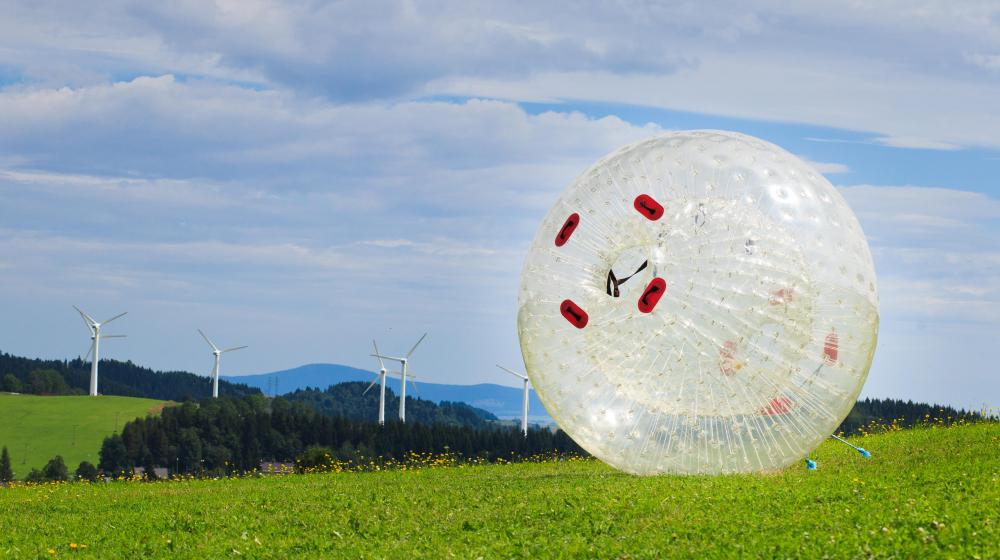# What is the Formula for Potential Energy? (with pictures)

Victoria Blackburn
Victoria BlackburnEnergy that is stored in an object is known as potential energy.

Potential energy is the energy that is stored in an object. Under the right conditions, this energy can be released as kinetic energy, or the energy of movement. Many different types of objects have energy stored as potential energy, including fuel, food, and springs. The potential energy of an object can be calculated using the formula for potential energy.Swinging on a swing is a good example of both potential and kinetic energy.

There are different kinds of potential energy based on how the energy is stored. Food and fuel contain chemical potential energy, while stretched elastic bands have elastic potential energy. The formula for potential energy that is used depends on the type of energy that is stored. In this article, the formula that is discussed is for gravitational potential energy. Gravitational potential energy is the potential energy of an object due to the height of the object above some reference point, which is usually the Earth’s surface.The formula for potential energy that is used depends on the type of energy that is stored.

To calculate the potential energy of an object, the formula for potential energy (PE) is PE = mgh, where m stands for mass of the object in kilograms (kg), g is the gravitational field strength and h is the height of the object in meters (m). Rarely is an object raised high enough to change the gravitational field, so 9.8 m/s2 (meters per second squared) is almost always used in the formula for potential energy. In some cases, GPE is used instead of PE to show that gravitational potential energy is being calculated.

For example, a 25 kg weight is raised 5 m above the ground. Compared to when it was resting on the ground, the change in potential energy can be calculated by substituting the numbers into the equation as such: PE = mgh = 25 x 9.8 x 5 = 1225 joules (J). Joules are the units used to measure energy and are named after a scientist named James Joule. In the mid 1800s, Joule performed a large number of experiments to prove that heat and energy were the really the same thing.

From the previous example, it was determined that the weight has 1225 J of stored energy, or potential energy. What this means is that if the weight were released, this stored energy would be converted to kinetic energy as it fell towards the ground. Energy is conserved — it is neither created nor lost. As a result, just before the weight hit the ground, its kinetic energy would be the same as its potential energy, or 1225 J.

## You might also LikeValleyFiah

@ Alchemy- To answer this, you need to use the following equation PE=mg[delta]h. You will also need to convert the grams into kilograms, and the number of feet into meters. Two hundred and eighty-seven grams is 0.287 kilograms. The change in height is 34 feet, which is the equivalent of 10.363 meters. Now just plug in your variables and solve the equation.

[delta]PE=0.287kg*9.80m/s^2*10.363m=29.15J. Your change in energy is 29.15 Joules.

Alchemy

How would I determine the change in potential energy of an object? The example of potential energy I was given is a 287 gram object is raised from 13 feet to 47 feet at sea level. What is the change in potential energy of the object?